# Basic Design of Flying Wing Models

## Preface

 A survey of the available literature on the topic of flying wing and tailless model airplanes shows, that in most cases, the airfoil selection is mentioned, but no reliable data for the selection are available. Due to the dedicated work of various modelers, a huge collection of airfoil coordinates is available today [UIUC], [Bender/Wiechers] - a comparable set of aerodynamic data is more difficult to find, though. This situation and, the numerous questions of modelers, concerning the performance and the selection of airfoils for flying wing models, initiated this paper. On this web page, you will find a way to perform the necessary calculations for stable flying wing models. The accompanying collection of airfoil coordinates and polars has been published in .An indoor flying wing model by R. Eppler in 1942.

## Airfoils for Flying Wings

 Tailless planes and flying wings can be equipped with almost any airfoil, if sweep and twist distribution are chosen accordingly. Thus, the one and only "flying wing airfoil" does not exist. However, if we want to design a tailless plane with a wide operating range, the wing should have a small amount of twist only, or none at all, to keep the induced drag at reasonable levels throughout the whole flight envelope. Under these conditions, the wing must not create a large variation in moment coefficient, when the angle of attack is varied. This makes it necessary, to use airfoils with a low moment coefficient. In the case of an unswept wing ("plank"), even an airfoil with a positive moment coefficient is necessary, to avoid upward deflected flaps under trimmed flight conditions. Such airfoils usually have a reflexed camber line.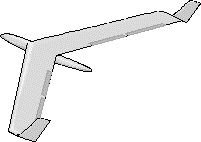A modern radio controlled F3B flying wing model of 1994.

## Longitudinal Stability

Like its full sized cousins, each model airplane should have a minimum amount of stability, i.e. it should be able to return to its trimmed flight condition after a disturbance by a gust or a control input. How much stability is required, depends on the pilots personal taste: contest pilots prefer a small stability margin, beginners like to fly with a large margin. Here, only a brief introduction into the topic will be given, which will make it possible to find a first guess for the center of gravity and a reasonable combination of sweep and camber for a flying wing.

### 1. Unswept Wings (Plank)

While the horizontal tailplane provides the necessary amount of longitudinal stability on a conventional plane, it is the wing, which stabilizes an unswept wing. In most cases, airfoils with reflexed (s-shaped) mean lines are used on flying wing models to achieve a longitudinally stable model.

#### Some important Aerodynamic and Mechanical Facts

To understand, why a reflexed airfoil is able to provide longitudinal stability to a wing, two things are important:

Total Force and Moment, c/4 point

The pressure forces, which act on the surface of each wing section, can be replaced by a single total force and a single total moment. Both act at the quarter-chord point of the airfoil. When the angle of attack changes (e.g. due to a gust), the moment stays nearly constant, but the total force changes. Increasing the angle of attack increases the force.

Center of Gravity

Translations and rotations of "free floating" bodies are performed relative to their center of gravity. When the angle of attack of a plane changes, the plane rotates (pitches) around its center of gravity (c.g.).

#### Equilibrium

Let's have a look at a trimmed flight condition, where all forces and moments are in equilibrium and let's compare a conventional, cambered airfoil with an airfoil with a reflexed camber line. The moments and forces for this trimmed state are denoted with an asterisk (*). The forces are the weight of the model m, multiplied with the gravity acceleration g (9.81m/s) and the aerodynamic lift L, which have to cancel out (sum of forces in vertical direction equals zero). The drag forces are neglected here. The sum of the moments around c.g. (caused by the airfoil moment M and the lift force L, acting at a distance from c.g.) must also be zero.

conventional airfoil with camber airfoil with reflexed mean line
Equilibrium State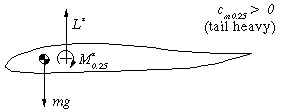This airfoil has a nose heavy moment. As stated above, the center of gravity is also the center of rotation of the wing. When it is shifted behind the c/4 point, the air force L* in front of the c.g. counteracts the nose heavy moment M* to achieve equilibrium. The distance between c.g. and c/4 point is depending on the amount of M*. A symmetrical airfoil has M*=0, which means we have to place the c.g. at the c/4 point. The reflexed camber line makes the moment coefficient positive, which means, that the moment around the c/4 point is working in the tail heavy direction. Therefore the center of gravity has to be located in front of the c/4 point to balance the moment M* by the lift force L*. The larger the moment (-coefficient) of the airfoil, the larger the distance between c/4 and the c.g. for equilibrium.
Disturbed State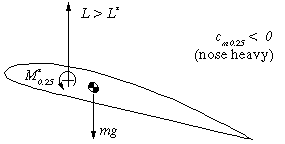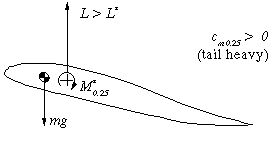When the angle of attack is increased (e.g. by a gust), the lift force L increases. Now L>L* and the tail heavy moment due to the lift is larger than the moment around c/4, which still is M=M*. Thus the wing will pitch up, increasing the angle of attack further. This behavior is instable and a tailplane is needed to stabilize the system. Here, we have the air force acting behind the c.g., which results in an additional nose heavy moment, when the lift increases. With L>L*, the wing will pitch down, reducing the angle of attack, until the equilibrium state is reached again. The system is stable.

#### Neutral Point and Stability

As we learned above, an unswept wing with a reflexed airfoil is able to stabilize itself. Its c.g. must be located in front of the c/4 point, which is also called neutral point (n.p.). The distance between the neutral point (quarter chord point for an unswept wing) and the center of gravity is defining the amount of stability - if the c.g. is close to the n.p., the straightening moment is small and the wing returns (too) slowly into its equilibrium condition. If the distance c.g. - n.p. is large, the c.g. is far ahead of the c/4 point and the wing returns quickly to the equilibrium angle. You will require larger flap deflections to control the model, though. If the distance is too large, the wing may become over-stabilized, overshooting its trimmed flight attitude and oscillating more and more until the plane crashes.

A measure for stability is the distance between c.g. and n.p., divided by the mean chord of the wing. Typical values for this number for a flying wing are between 0.02 and 0.05, which means a stability coefficient sigma of 2 to 5 percent. We can express the equilibrium of moments around c.g. for our design lift coefficientby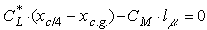,

which can be transformed to find the moment coefficient needed to satisfy a certain stability coefficient: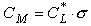.

Example We want to use an unswept flying wing (a plank) for ridge soaring and decide to use a target lift coefficient of=0.5. We want to have a stability coefficient of 5% and are looking for a matching airfoil. We calculate the necessary moment coefficient Cm = 0.5 * 0.05 = +0.025. Searching through a publication about Eppler airfoils , we find, that the airfoils E 186 and E 230 could be used for our model.

### 2.1 Neutral Point and Stability

We have already learned, that the center of gravity must be located in front of the neutral point. While the n.p. of an unswept, rectangular wing is approximately at the c/4 point, the n.p. of a swept, tapered wing must be calculated. The following procedure can be used for a simple, tapered and, swept wing. First, we calculate the mean aerodynamic chord length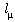of a tapered wing, which is independent from the sweep angle: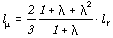with the root chord lr, the tip chord lt and the taper ratio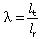.

We can also calculate the spanwise location of the mean chord, using the span b,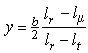.

The n.p. of our swept wing can be found by drawing a line, parallel to the fuselage center line, at the spanwise station y. The chord at this station should be equal to. The n.p. is approximately located at the c/4 point of this chord line (see the sketch below).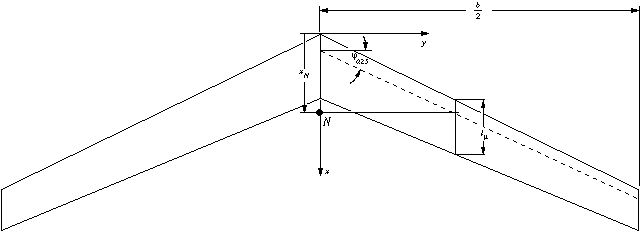Geometric parameters of a tapered, swept wing.

Instead of using the graphical approach, the location of the neutral point can also be calculated by using one of the following formulas, depending on the taper ratio: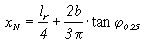, if taper ratio > 0.375, if taper ratio < 0.375.

The c.g. must be placed in front of this point, and the wing may need some twist (washout) to get a sufficiently stable wing.

### 2.2 Twist

The selection of the location of the c.g. to be infront of the n.p.  is not a guarantee for equilibrium - it is only a requirement for longitudinal stability. Additionally, as explained above for unswept wings, the sum of all aerodynamic moments around the c.g. must be zero. Because we have selected the position of the c.g. already to satisfy the stability criterion (c.g. in front of n.p.), we can achieve the equilibrium of the moments only by airfoil selection and by adjusting the twist of the wing. On conventional airplanes with a horizontal stabilizer it is usually possible to adjust the difference between the angles of incidence of wing and tailplane during the first flight tests. On the other hand, flying wings have the difference built into the wing (twist), which cannot be altered easily. Thus it is very important to get the combination of planform, airfoils and twist right (or at least close) before the wing is built. Again, the calculation of these parameters is quite complex and shall not be presented here; the relations are shown in great detail in . Here I will present a simple, approximate approach, which is based on two graphs, and can be used for swept, tapered wings with a linear airfoil variation from root to tip.

We start with the same geometric parameters, which we have used for the calculation of the n.p. above. Additionally, we calculate the aspect ratio (AR = b²/S, where S is the wing area) of the wing. The selection of the airfoil sections also defines the operating range of the model. Airfoils with a small amount of camber are not well suited for slow, thermaling flight, but good for F3B flight style and ridge soaring. We can design the twist distribution for one trimmed lift coefficient, where the wing will fly without flap deflections. This lift coefficient will usually be somewhere between the best glide and the best climb performance of the airfoil. With the selected lift coefficient Cl of the airfoils, we can also find the moment coefficient Cm0.25 from the airfoil polars. If we plan to use different root and tip sections, we use the mean value of the moment coefficient of the two airfoils. The required twist of the wing can be combined from two parts:

Geometric Twist
This is the twist, which is built into the wing as the difference between the x-axis of the root and the tip section. It corresponds to the angle difference between main wing and tailplane of conventional planes and can be easily measured. A positive twist means a smaller angle of incidence at the tip section (washout). Large geometric twist angles can be used to stabilize wings with small sweep angles or highly cambered airfoils, but have the drawback of creating large amounts of induced drag, when the wing is operated outside of its design point. The aim of the following paragraphs is to find the geometric twist.

Aerodynamic Twist
If we select airfoils with different zero lift angles, we can reduce the amount of geometric twist. The difference between the zero lift directions is called aerodynamic twist and we need airfoil polars to find the zero lift angle. Also, a small or even positive moment coefficient reduced the required amount of geometric twist, and improves the off design performance of the wing.

#### Finding the Required Twist ßreq

Using graph 1, we enter the graph with the aspect ratio AR on the horizontal axis, and draw a vertical line upwards, until we intersect the curve, corresponding to the sweep angle of the c/4 line. Continuing to the axis on the left border, we find the standard value b*req for the required twist angle.
This standard value is valid for a wing, which:

• is trimmed at= 1.0 and,

• has a stability coefficient of s* =10% (see above), and

• uses airfoils with a moment coefficient of zero.

From the standard value we calculate the true, required twist angle, using the formula inset into the graph. Therefore, we calculate the ratio of our target lift coefficient to the standard lift coefficient (CL/) and the ratio of our desired stability coefficient to the standard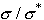. We see, that a reduction of the lift coefficient to CL=0.5 also reduces the required twist by 50%. Also, if we use a smaller stability margin s, we need a smaller amount of twist.Graph 1: Finding the required twist.

#### Variation of zero lift angle

If we use different airfoils at root and tip, they may have different zero lift directions, which influences the equilibrium state. The geometric twist has to be reduced by the difference of the zero lift directions a0 of tip and root sections:.

Using the same airfoil for both sections, we can set ba0 to zero.

#### Influence of the Airfoil Moment coefficients

The moment coefficient of the airfoils contributes to the equilibrium, and has to be taken into account for the calculation of the twist. Graph 2 can be used to find the equivalent twist due to the contribution of Cm, which has to be subtracted from the required twist. If we use airfoils with positive moment coefficients, the contribution will be positive, which results in a reduction of the amount twist, highly cambered airfoils yield negative values bCm, which force us to build more twist into the wing. Similar to the previous graph, we enter with the aspect ratio, intersect with the sweep curve and read the value for b*Cm from the lefthand axis.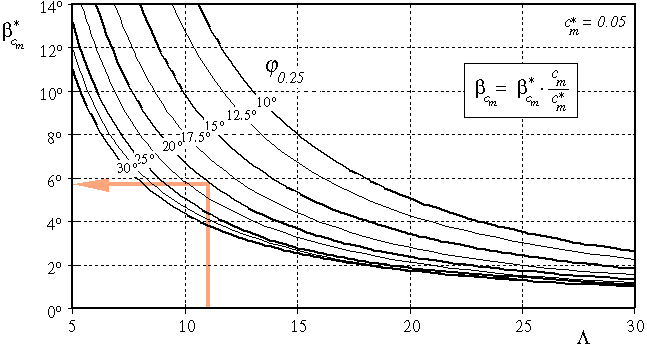Graph 2: Finding the additional twist due to the airfoils moment coefficient.

Again, the graph has been plotted for a certain standard condition, which is a moment coefficient of cm* = 0.05 (note: positive value). We apply the ratio of the moment coefficients (cm/cm*) to find the contribution bCm of the moment coefficient to the geometric twist. This contribution has to be subtracted from the required twist angle, too. Using the usual, cambered airfoils with negative moment coefficients will change the sign of the ratio cm/cm*, which results in negative b*Cm values. This means, that the subtraction from breq will actually be an addition, increasing the geometric twist angle. If we have different airfoils at root and tip, we can use the mean moment coefficient (cm,tip + cm,root)/2 to calculate the ratio cm/cm*.

Finally, we can calculate the geometric twist angle bgeo, which has to be built into the wing: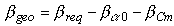.

Example As you have noticed, the graphs contain an example, which is used here. We consider a flying wing model with the following data:
 wing span b = 2.365 m chord length at root lr = 0.260 m chord length at tip lt = 0.170 m sweep angle at c/4 line j0.25 = 20° design lift coefficient CL = 0.5 root section E 182 cm,r = +0.01   and   a0,r = -0.3° tip section E 184 cm,t = +0.03   and   a0,t = 0.5° desired stability coefficient sigma = 0.05

We calculate the wing area S:

S = (l_r + l_t)/2 * b = 0.5085 m²

and the aspect ratio

AR = b²/S = 11.0

and the mean moment coefficient

cm = (cm,r + cm,t)/2 = 0.02 .

Using graph 1, we find b*req = 11.8°, which has to be corrected to match our design lift coefficient and the desired stability margin: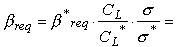11.8 * (0.5/1.0) * (0.05/0.1) = 2.95° .

This means that our model would need a twist angle of 2.95° (wash out) from root to tip, if we would use a symmetrical airfoil section.

The difference of the zero lift angle of tip and root section is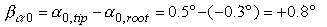.

Now we read the twist contribution of the moment coefficient from graph 2, which is b*Cm = 5.8°, which has to be corrected for our smaller mean moment coefficient: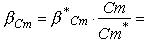5.8 * (0.02/0.05) = 2.32° .

Finally, we calculate the geometric twist from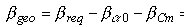2.95° - 0.8° - 2.32° = -0.17° .

The negative value means, that we could use a small amount of wash-in! This is because we have already enough stability due to the selection of airfoils with reflexed camber lines. Since the calculated amount is very small, we can use the same angle of incidence for the root and tip ribs. Since the presented method is not perfect, we can assume an accuracy to 1 degree, which is also a reasonable assumption for the average building skills.Due to the increasing amount of SPAM mail, I have to change this e-Mail address regularly. You will always find the latest version in the footer of all my pages.

It might take some time until you receive an answer and in some cases you may even receive no answer at all. I apologize for this, but my spare time is limited. If you have not lost patience, you might want to send me a copy of your e-mail after a month or so.
This is a privately owned, non-profit page of purely educational purpose. Any statements may be incorrect and unsuitable for practical usage. I cannot take any responsibility for actions you perform based on data, assumptions, calculations etc. taken from this web page.